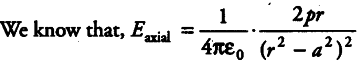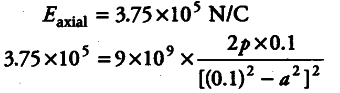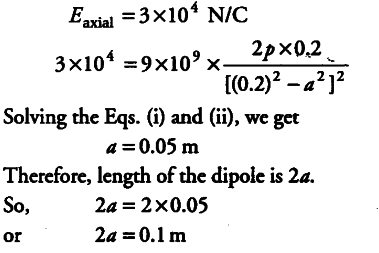# Calculate the length of an electric dipole

The electric field at a point on the axial line at a distance of 10 cm from the centre of an electric dipole is 3.75x\${{10}^{5}}\$ N/C in air, while at a distance of 20 cm, the electric field is 3 x \${{10}^{4}}\$ N/C. Calculate the length of an electric dipole.Case 1,
When r = 10cm , r =0.1mCase II, When r = 20 cm = 0.2 m